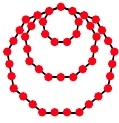# C program to find Decagonal Number?

CServer Side ProgrammingProgramming

A decagonal number is a figurate that was derived using the concept of triangular numbers and square numbers. This extension of number pattern is created using non-rotationally symmetrical numbers. This nested decagon oriented number is given by the number of dots in the number of nested decagons that are created.

For example, for 3rd decagonal number 3 decagonal figures are nest each with iX time the number of sides in the decagon. As shown in figure −Side in 1st Outer figure = 30

Side in 2nd Outer figure = 20

Side in inner figure = 10

Sides common in all = 6+2

Total = 30+20+10-(6+2) = 52

This result is generalized using the formula 4n2 - 3n.

This given the decagonal number for n